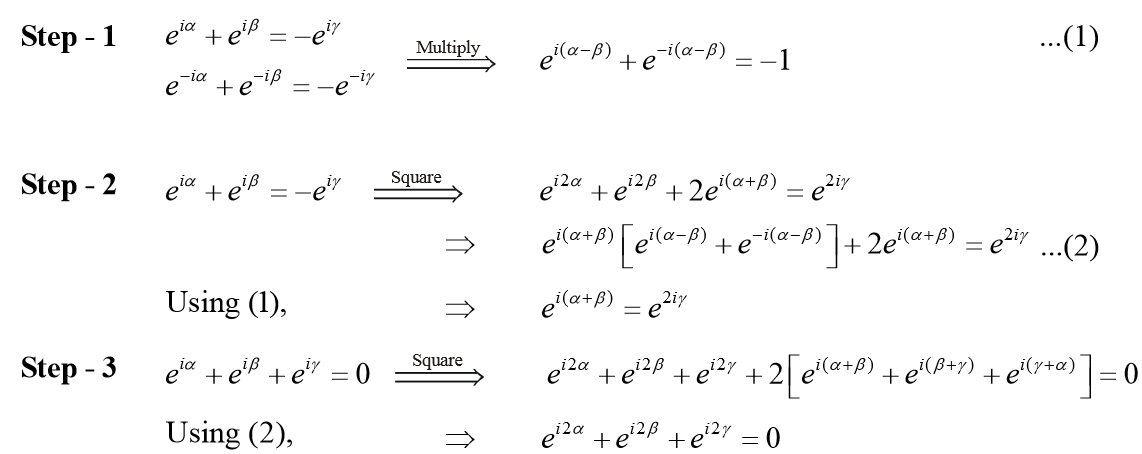# Examples on Trigonometric Ratios and Functions Set 3

Go back to  'Trigonometry'

Example-8

Let  $$\cos \alpha +\cos \beta +\cos \gamma =\sin \alpha +\sin \beta +\sin \gamma =0$$ . Evaluate

(a) $${{\cos }^{2}}\alpha +{{\cos }^{2}}\alpha +{{\cos }^{2}}\gamma$$
(b) $${{\sin }^{2}}\alpha +{{\sin }^{2}}\beta +{{\sin }^{2}}\gamma$$

Solution: It should be evident to you that if we are able to evaluate part - (a), the answer to part-(b) is automatically determined since  $${{\cos }^{2}}\alpha =1-{{\sin }^{2}}\alpha$$ and so on.
Now, we proceed to evaluate part - (a):

\begin{align} & \cos \alpha +\cos \beta =-\cos \gamma \,\,\,\,\,\,\,\,\,\,\,\,\,\,\,\,\,\,...\left( 1 \right) \\ & \sin \alpha +\sin \beta =-\sin \gamma \,\,\,\,\,\,\,\,\,\,\,\,\,\,\,\,\,\,\,\,\,...\left( 2 \right) \\ \end{align}

$2+2\cos (\alpha -\beta )=1\Rightarrow \cos (\alpha -\beta )=-\frac{1}{2}$

Similarly,

$\cos (\beta -\gamma )=-\frac{1}{2},\ \ \cos (\gamma -\alpha )=-\frac{1}{2}$

Now, if we square (1) and (2) and subtract, we have

\begin{align}& \;\;\;\,\,\,\,\,\,\,\,\,\,\,\,\,\,\,\,\cos 2\alpha +\cos 2\beta +2\cos (\alpha +\beta )=\cos 2\gamma \\ & \Rightarrow\qquad 2\cos \left( \alpha +\beta \right)\cos \left( \alpha -\beta \right)+2\cos \left( \alpha +\beta \right)=\cos 2\gamma \\\end{align}

Since
\begin{align} & \cos (\alpha -\beta )=-\frac{1}{2},\ \text{we}\ \text{have} \\& \cos (\alpha +\beta )=\cos 2\gamma \,\,\,\,\,\,\,\,\,\,\,\,\,\,\,\,\,\,\,\,\,\,\,\,\,\,\,\,\,\,\,\,\,\,\,\,\,\,\,\,\,\,\,\,\ldots \left( 3 \right) \\\end{align}

and similarly, two other relations
Now,
\begin{align}&\;\; \,\,\,\,\,\,\,\,\,\,\,\,\,\,\,\,{{(\cos \alpha +\cos \beta +\cos \gamma )}^{2}}-{{(\sin \alpha +\sin \beta +\sin \gamma )}^{2}}=0 \\ & \Rightarrow\qquad \cos 2\alpha +\cos 2\beta +\cos 2\gamma +2[\cos (\alpha +\beta )+\cos (\beta +\gamma )+\cos (\gamma +\alpha )]=0 \\\end{align}

Using (3), this reduces to

$\cos 2\alpha +\cos 2\beta +\cos 2\gamma =0$

Since $$\cos 2\alpha =2{{\cos }^{2}}\alpha -1$$  and so on, we finally have

${{\cos }^{2}}\alpha +{{\cos }^{2}}\beta +{{\cos }^{2}}\gamma =\frac{3}{2}$

This further implies that

${{\sin }^{2}}\alpha +{{\sin }^{2}}\beta +{{\sin }^{2}}\gamma =\frac{3}{2}$

Alternative:

An equivalent solution can be presented very effectively using complex numbers. Recall the Euler’s form of a complex number:

${e^{i\theta }} = \cos \theta + i\sin \theta$

Using the Euler’s form, the given relations reduce to

\begin{align} & {{e}^{i\alpha }}+{{e}^{i\beta }}+{{e}^{i\gamma }}=0 \\ & {{e}^{-i\alpha }}+{{e}^{-i\beta }}+{{e}^{-i\gamma }}=0 \\ \end{align}

The solution is now 3 steps away:Finally, we take the real parts:

\begin{align}&\quad\,\,\,\,\,\,\,\,\,\,\,\,\,\cos 2\alpha +\cos 2\beta +\cos 2\gamma =0 \\ & \Rightarrow \qquad {{\cos }^{2}}\alpha +{{\cos }^{2}}\beta +{{\cos }^{2}}\gamma =\frac{3}{2} \\\end{align}

For students comfortable with complex numbers, most of the steps presented here can be done mentally.

Example-9

Find the range of  $$f(x)={{\sin }^{2}}x+{{\cos }^{4}}x$$

Solution: A calculus approach

\begin{align} & f'(x)=2\sin x\cos x-4{{\cos }^{3}}x\sin x \\ & \,\,\,\,\,\,\,\,\,\,\,\,\;=2\sin x\cos x(1-2{{\cos }^{2}}x)=\sin 2x(1-2{{\cos }^{2}}x) \\ \end{align}

The critical points in \begin{align}\left[ 0,\ \frac{\pi }{2} \right],\ \text{are}\ x=0,\frac{\pi }{4}\end{align}   which implies

\begin{align} & {{f}_{\text{max}}}(x)=f(0)=1, \\ & {{f}_{\min }}(x)=f\left( \frac{\pi }{4} \right)=\frac{3}{4} \\ \end{align}

A trigonometric approach

\begin{align} & f(x)={{\cos }^{4}}x-{{\cos }^{2}}x+1 \\ & \,\,\,\,\,\,\,\,\,\,\,={{\left( {{\cos }^{2}}x-\frac{1}{2} \right)}^{2}}+\frac{3}{4}\ge \frac{3}{4}\quad\Rightarrow \qquad\;\;\;{{f}_{\min }}(x)=\frac{3}{4} \\ & f(x)={{\cos }^{2}}x({{\cos }^{2}}x-1)+1 \\ & \,\,\,\,\,\,\,\,\,\,\,=1-{{\sin }^{2}}x{{\cos }^{2}}x\le 1\qquad\qquad\Rightarrow \qquad{{f}_{\max }}(x)=1 \end{align}